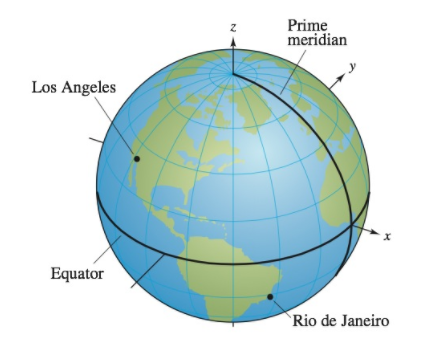Chapter 11, Problem 16PS

Chapter
Section
Textbook Problem

# Latitude-Longitude SystemLos Angeles is located at 34.05° North latitude and 118.24° West longitude, and Rio de Janeiro, Brazil, is located at 22.90° South latitude and 43.23° West longitude (see figure). Assume that Earth is spherical and has a radius of 4000 miles.(a) Find the spherical coordinates for the location of each city.(b) Find the rectangular coordinates for the location of each city.(c) Find the angle (in radians) between the vectors from the center of Earth to the two cities.(d) Find the great-circle distance s between the cities. (Hint: s = r θ )(e) Repeat parts (a)–(d) for the cities of Boston, located at 42.36° North latitude and 71.06° West longitude, and Honolulu, located at 21.31° North latitude and 157.86° West longitude.

(a)

To determine

To calculate: The spherical coordinates for the location of cities Los Angeles and Rio de Janeiro.

Explanation

Given: Los Angeles is located at 34.05° North latitude and 118.24° West longitude, and Rio de Janeiro is located at 22.90° South latitude and 43.23° West longitude. Assume that Earth is spherical and has a radius of 4000 miles.

For thefigure refer to the question.

Formula used: In a spherical coordinate system, a point P in space is represented by an ordered triple (ρ,θ,ϕ) where ρ is the distance between origin and the point, θ and ϕ are the angles between line segment from origin towards the point and the positive axes-x and z respectively.

Calculation: Let the provided city be a point on Earth and the distance between the origin of Earth and the city is equal to the radius of Earth.

Therefore, ρ=4000

Since, 118.24° clockwise from prime meridian. Therefore,

θ=118.24°

The latitude is above equator

(b)

To determine

To calculate: The rectangular coordinates for the location of cities: Los Angeles and Rio de Janeiro.

(c)

To determine

To calculate: The angle (in radians) between the vectors from the center of Earth to the two cities.

(d)

To determine

To calculate: The great circle distance s between the cities: Los Angeles and Rio de Janeiro.

(e)

To determine

To calculate: The spherical and rectangular coordinates for the cities: Boston and Honolulu, the angle between the vectors from the center of Earth to the two cities, and the great circle distance s between them.

### Still sussing out bartleby?

Check out a sample textbook solution.

See a sample solution

#### The Solution to Your Study Problems

Bartleby provides explanations to thousands of textbook problems written by our experts, many with advanced degrees!

Get Started